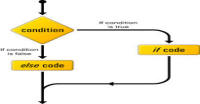# Conditional Statements in Ruby

What will we do if we want to execute code only in a specific condition? The conditional statements help us here. Lets take an example, I want to check if a number is in range 0 to 100 or not.

### If statement

This check a condition and if condition is true then executes the code inside it.

```print 'give a number: '
# take a number from user
# execute this code and write a number
a = gets.to_i

# check condition
if a < 100
puts 'a is less than 100'
end
```

### If else statement

This check a condition and if condition is true then execute the code inside it otherwise execute the code inside the else statement.

```print 'give a number: '
# take a number from user
# execute this code and write a number
a = gets.to_i

# check condition
if a < 100 and a >0
puts 'a is between 0 and 100'
else
# code inside else statement
puts 'a is outside the range'
end
```

### If and elsif statement:

This statement check one condition if condition is false then check condition of elsif and it condition is true then run code inside that statement otherwise continue to next condition.

```print 'give a number: '
# take a number from user
# execute this code and write a number
a = gets.to_i

# if condition
if a < 100 and a > 0
puts 'a is between 0 and 100'
# elsif condition
elsif a<1000
puts 'a is less than 1000'
# statement can has multiple elsif statement
# finally else statement
else
puts 'a is out of range'
end
```

### If modifier:

This is modified one line if statement. Syntax is => code if condition

```print 'give a number: '
# take an integer from user
# execute this code and write a number
a = gets.to_i

# if modifier statement
puts 'a is less than 100' if a<100
```

### Ruby unless statement:

This is opposite to the if statement. The code inside this statement executes when condition is false. You can assume it as an if statement with opposite condition.

```print 'give a number: '
# take an integer from user
# execute this code and write a number
a = gets.to_i

# unless statement
unless a>= 100
puts 'a is less than 100'
end
```

### Ruby unless modifier:

This is modified one line unless statement.

```print 'give a number: '
# take an integer from user
# execute this code and write a number
a = gets.to_i

# modified unless statement
puts 'a is less than 100' unless a>= 100
```

### Ruby case statement:

Case statement is a modified if elsif statement, in which we take a data value and compare that value with a number of cases.

```print 'give a number: '
# take an integer from user
# execute this code and write a number
a = gets.to_i

# take a data value using keyword case
case a
# check conditions => 1..99 means in the range of 1 to 99
when 1..99
puts 'a is between 0 and 100'
# check from 100 to 1000
when 100..1000
puts 'a is between 99 and 1000'
# if above two cases fail then use else statement
else
puts 'a is outside the range'
# close case using end keyword
end
```

#### Click on banner below to Learn: PHP with Laravel for beginners - Become a Master in LaravelHarish, a technical core team member at www.lyflink.com with five year experience in full stack web and mobile development, spends most of his time on coding, reading, analysing and curiously following businesses environments. He is a non-graduate alumni from IIT Roorkee, Computer Science and frequently writes on both technical and business topics.

### Related ArticlesPHP stands for PHP: Hypertext Preprocessor. PHP is known as a server side scripting and interpreter language for web ...
##### Introduction to PHPIn this tutorial, we will study about conditional statements (if else statements and switch statements). These statem...
##### Conditional statements in phpIn this tutorial, we will study about strings and operations upon strings. Due to long length of this section, I have...

### The Complete Web Developer Course - Build 25 Websites